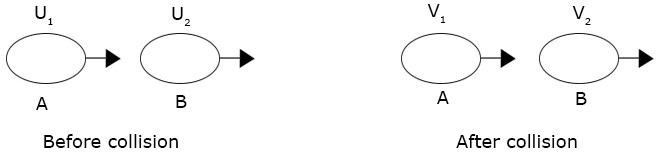### Linear momentum II

Physics SSS2 Second Term

Sub Theme: Conservation Principle

WEEK 2

Linear momentum II

Performance Objectives

Students should be able to;

1. Distinguish between elastic and inelastic collision.
2. Explain inertia, inertia mass and weight, and the relationship between mass and weight.
3. Explain why walking is possible.
4. Explain why the weight of a body may vary from place to place.

The Principle of Conservation of Linear Momentum

Content

In a closed system of colliding bodies, the total momentum after the collision is equal to the total momentum before the collision provided there is no net external force acting on the system.

Case 1: Consider two bodies A and B of masses m1 and m2 moving in the same direction with velocities u1 and u2 respectively. After collision their velocities were V1 and V2 as shown below.View More